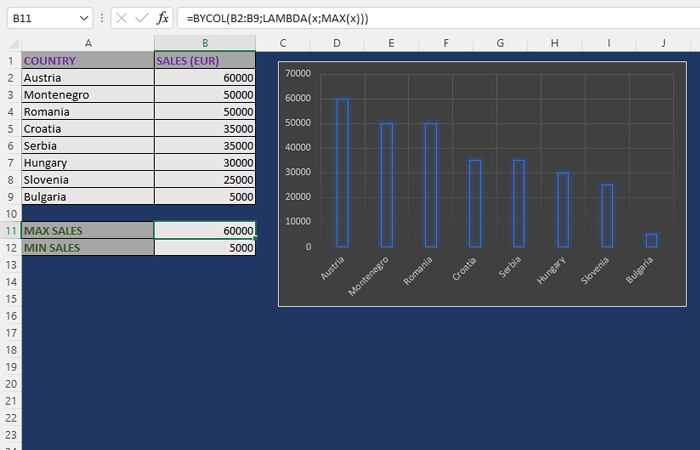# BYROW and BYCOL functions

The BYROW and BYCOL functions are used to perform a calculation given by a LAMBDA function that uses only one argument for a given row or column. This, in a way, distinguishes them from the function mentioned in the previous post. Both functions work in a similar way. They differ only in whether they retrieve the data needed for the calculation from the row or column.

The syntax of these functions looks like this:

BYROW (<array>,<calculation>)

BYCOL (<array>,<calculation>)

For example, if the table shows an overview of sales by country using the formula:

=BYCOL(B2:B9,LAMBDA(x,MAX(x)))

we calculate the maximum value of sales. If we want to reach the minimum value of sales, we will write the formula:

=BYCOL(B2:B9,LAMBDA(x,MIN(x)))

If, in some cases, the data were in rows, we would write similar formulas. The only difference is that we would then use the BYROW function.